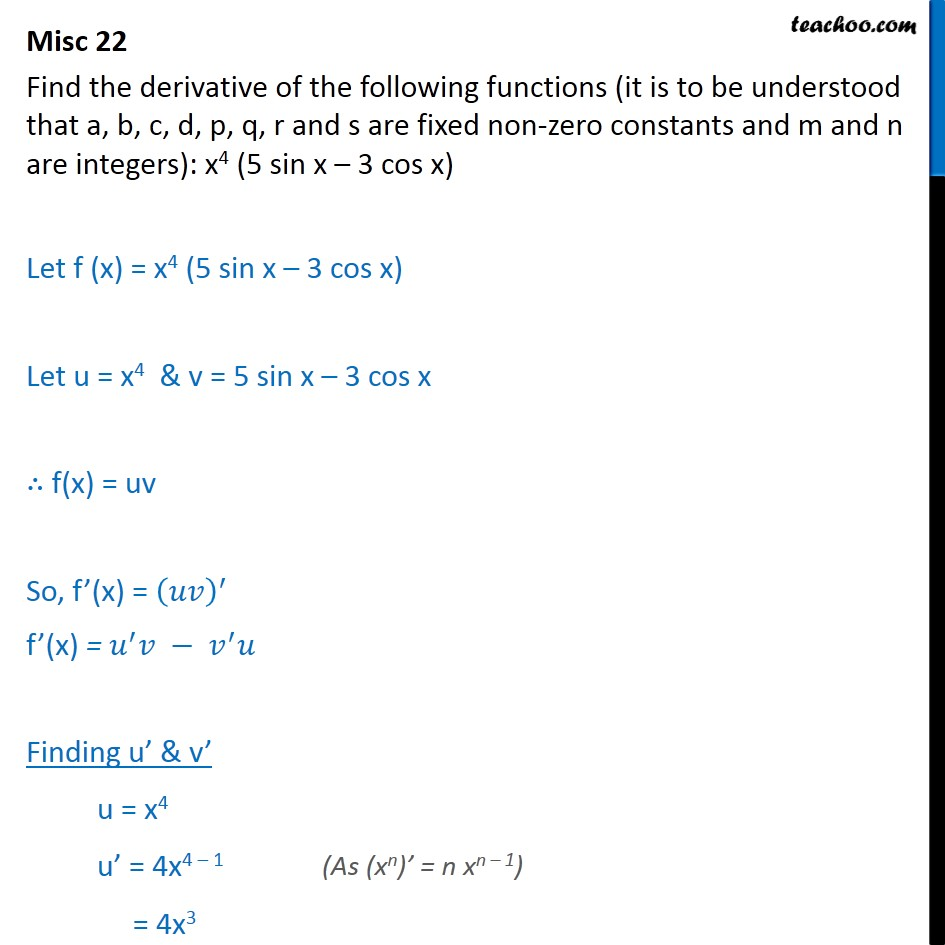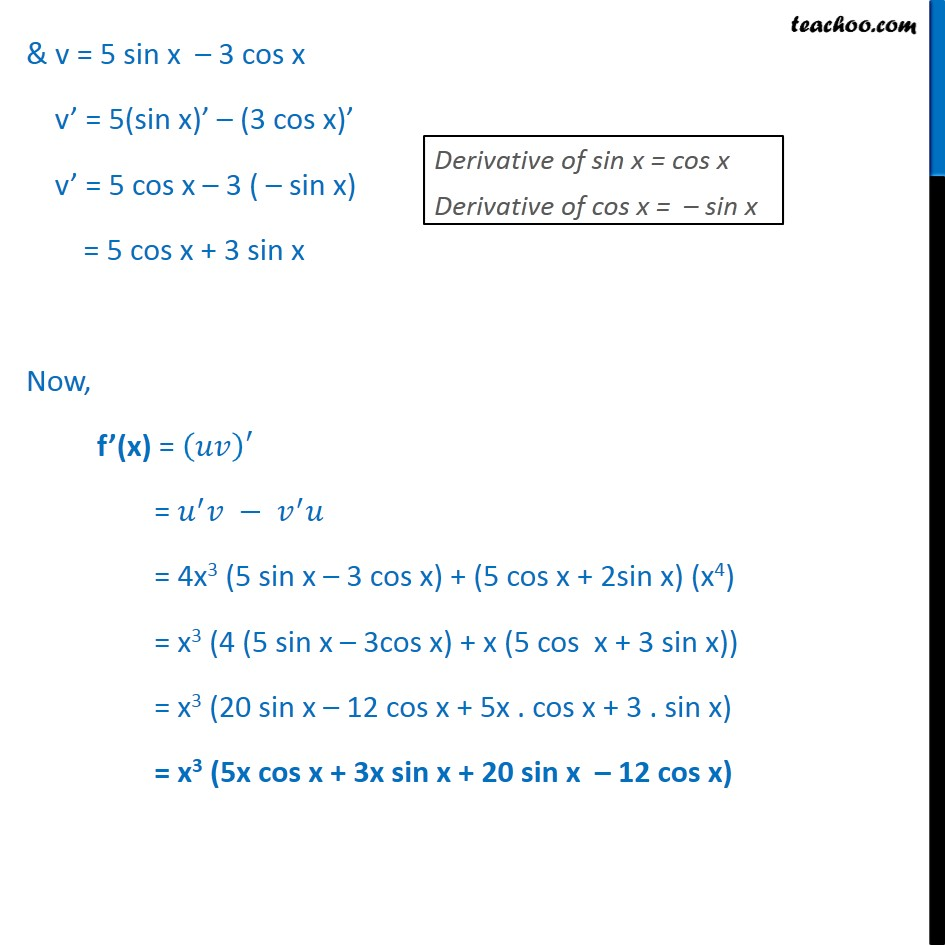Derivatives by formula - sin & cos

Chapter 12 Class 11 Limits and Derivatives
Concept wiseLearn in your speed, with individual attention - Teachoo Maths 1-on-1 Class

### Transcript

Misc 22 Find the derivative of the following functions (it is to be understood that a, b, c, d, p, q, r and s are fixed non-zero constants and m and n are integers): x4 (5 sin x – 3 cos x) Let f (x) = x4 (5 sin x – 3 cos x) Let u = x4 & v = 5 sin x – 3 cos x ∴ f(x) = uv So, f’(x) = 𝑢𝑣﷯﷮′﷯ f’(x) = 𝑢﷮′﷯𝑣 − 𝑣﷮′﷯𝑢 Finding u’ & v’ u = x4 u’ = 4x4 – 1 = 4x3 & v = 5 sin x – 3 cos x v’ = 5(sin x)’ – (3 cos x)’ v’ = 5 cos x – 3 ( – sin x) = 5 cos x + 3 sin x Now, f’(x) = 𝑢𝑣﷯′ = 𝑢﷮′﷯𝑣 − 𝑣﷮′﷯𝑢 = 4x3 (5 sin x – 3 cos x) + (5 cos x + 2sin x) (x4) = x3 (4 (5 sin x – 3cos x) + x (5 cos x + 3 sin x)) = x3 (20 sin x – 12 cos x + 5x . cos x + 3 . sin x) = x3 (5x cos x + 3x sin x + 20 sin x – 12 cos x)